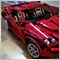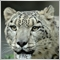# Problem coding my indicator, HELP PLZE14

Hi, im trying to code a basic program with mql4 but since im a beginner, i don't really know how to code and i've got some problems, i don't even know if my program is okay.

when price break the upper side of a rectangle which represent a zone, the indicator should send me an alert when price does a retest at this same side of the rectangle. i wrote this code but there are somes errors and i don't know if it's good. if someone can give me some advices, it would be nice. thank you

```//+------------------------------------------------------------------+
//|                                                       tnaahn.mq4 |
//|                                                           tnaahn |
//|                                             https://www.mql5.com |
//+------------------------------------------------------------------+
#property copyright "tnaahn"
#property link      "https://www.mql5.com"
#property version   "1.00"
#property strict
#property indicator_chart_window
//--- input parameters

//--- variables
double Vask = MarketInfo (NULL, MODE_ASK);
double Vbid = MarketInfo (NULL, MODE_BID);
double close;
int barre = 1;
double objectget1 (
string OBJ_RECTANGLE,
int OBJPROP_PRICE1);
double objectget2 (
string OBJ_RECTANGLE,
int OBJPROP_PRICE2);

//+------------------------------------------------------------------+
//| Custom indicator initialization function                         |
//+------------------------------------------------------------------+
int init()
{
//---- indicators

//----
return(0);
}
//+------------------------------------------------------------------+
//| Custom indicator deinitialization function                       |
//+------------------------------------------------------------------+
int deinit()
{
//----

//----
return(0);
}
//+------------------------------------------------------------------+
//| Custom indicator iteration function                              |
//+------------------------------------------------------------------+
int start()
{

if (iClose(NULL,PERIOD_H4, barre) > objectget2)
{ if (Vask < objectget2)
SendNotification (OBJPROP_SYMBOL);
}
if (iClose(NULL,PERIOD_H4,barre) < objectget1)
{ if (Vbid > objectget1)
SendNotification (OBJPROP_SYMBOL)
}

{
return(0);
}
//+--------------------------------------------------------------function                         |
```

And here are the errors i've got:

'tnaahn.mq4'    tnaahn.mq4

'}' - unexpected end of program
'{' - unbalanced parentheses
OnCalculate function not found in custom indicator
3 error(s), 0 warning(s)410

tnaahn:

And here are the errors i've got:

'tnaahn.mq4'    tnaahn.mq4

'}' - unexpected end of program
'{' - unbalanced parentheses
OnCalculate function not found in custom indicator
3 error(s), 0 warning(s)

As the error said - you have problem with your {}. So if you just check through to make sure all { has a corresponding }, you'll see that the following is unnecessary:

```{
return(0);
}```

Next, these lines must be within the start() function, since you want them to be updated each time start() is triggered:

```double Vask = MarketInfo (NULL, MODE_ASK);
double Vbid = MarketInfo (NULL, MODE_BID);
```

Their declaration can remain global, if you want other functions to be able to access them.

Also, you declared these as functions, so you need to code their internals or else they won't know what to do:

```double objectget1 (
string OBJ_RECTANGLE,
int OBJPROP_PRICE1);
double objectget2 (
string OBJ_RECTANGLE,
int OBJPROP_PRICE2);
```

And since they are functions, you need to supply the variables they require when you call them - this explains the new compilation errors you'll see after you've remove the {.892

Hello friend,

you will need to resolve some other issues in your code, the following should resolve one.

```int OnCalculate(const int rates_total,
const int prev_calculated,
const datetime &time[],
const double &open[],
const double &high[],
const double &low[],
const double &close[],
const long &tick_volume[],
const long &volume[],
const int &spread[])
{
return(rates_total);
}```

Where you put this in most cases is important.

For your Object you want to create, please look  at this sample from the manual.

```#property strict
//--- description
#property description "Script creates rectangle on the chart."
#property description "Anchor point coordinates are set in"
#property description "percentage of the chart window size."
//--- display window of the input parameters during the script's launch
#property script_show_inputs
//--- input parameters of the script
input string          InpName="Rectangle"; // Rectangle name
input int             InpDate1=40;         // 1 st point's date, %
input int             InpPrice1=40;        // 1 st point's price, %
input int             InpDate2=60;         // 2 nd point's date, %
input int             InpPrice2=60;        // 2 nd point's price, %
input color           InpColor=clrRed;     // Rectangle color
input ENUM_LINE_STYLE InpStyle=STYLE_DASH; // Style of rectangle lines
input int             InpWidth=1;          // Width of rectangle lines
input bool            InpFill=true;        // Filling the rectangle with color
input bool            InpBack=false;       // Background rectangle
input bool            InpSelection=true;   // Highlight to move
input bool            InpHidden=true;      // Hidden in the object list
input long            InpZOrder=0;         // Priority for mouse click
//+------------------------------------------------------------------+
//| Create rectangle by the given coordinates                        |
//+------------------------------------------------------------------+
bool RectangleCreate(const long            chart_ID=0,        // chart's ID
const string          name="Rectangle",  // rectangle name
const int             sub_window=0,      // subwindow index
datetime              time1=0,           // first point time
double                price1=0,          // first point price
datetime              time2=0,           // second point time
double                price2=0,          // second point price
const color           clr=clrRed,        // rectangle color
const ENUM_LINE_STYLE style=STYLE_SOLID, // style of rectangle lines
const int             width=1,           // width of rectangle lines
const bool            fill=false,        // filling rectangle with color
const bool            back=false,        // in the background
const bool            selection=true,    // highlight to move
const bool            hidden=true,       // hidden in the object list
const long            z_order=0)         // priority for mouse click
{
//--- set anchor points' coordinates if they are not set
ChangeRectangleEmptyPoints(time1,price1,time2,price2);
//--- reset the error value
ResetLastError();
//--- create a rectangle by the given coordinates
if(!ObjectCreate(chart_ID,name,OBJ_RECTANGLE,sub_window,time1,price1,time2,price2))
{
Print(__FUNCTION__,
": failed to create a rectangle! Error code = ",GetLastError());
return(false);
}
//--- set rectangle color
ObjectSetInteger(chart_ID,name,OBJPROP_COLOR,clr);
//--- set the style of rectangle lines
ObjectSetInteger(chart_ID,name,OBJPROP_STYLE,style);
//--- set width of the rectangle lines
ObjectSetInteger(chart_ID,name,OBJPROP_WIDTH,width);
//--- enable (true) or disable (false) the mode of filling the rectangle
ObjectSetInteger(chart_ID,name,OBJPROP_FILL,fill);
//--- display in the foreground (false) or background (true)
ObjectSetInteger(chart_ID,name,OBJPROP_BACK,back);
//--- enable (true) or disable (false) the mode of highlighting the rectangle for moving
//--- when creating a graphical object using ObjectCreate function, the object cannot be
//--- highlighted and moved by default. Inside this method, selection parameter
//--- is true by default making it possible to highlight and move the object
ObjectSetInteger(chart_ID,name,OBJPROP_SELECTABLE,selection);
ObjectSetInteger(chart_ID,name,OBJPROP_SELECTED,selection);
//--- hide (true) or display (false) graphical object name in the object list
ObjectSetInteger(chart_ID,name,OBJPROP_HIDDEN,hidden);
//--- set the priority for receiving the event of a mouse click in the chart
ObjectSetInteger(chart_ID,name,OBJPROP_ZORDER,z_order);
//--- successful execution
return(true);
}
//+------------------------------------------------------------------+
//| Move the rectangle anchor point                                  |
//+------------------------------------------------------------------+
bool RectanglePointChange(const long   chart_ID=0,       // chart's ID
const string name="Rectangle", // rectangle name
const int    point_index=0,    // anchor point index
datetime     time=0,           // anchor point time coordinate
double       price=0)          // anchor point price coordinate
{
//--- if point position is not set, move it to the current bar having Bid price
if(!time)
time=TimeCurrent();
if(!price)
price=SymbolInfoDouble(Symbol(),SYMBOL_BID);
//--- reset the error value
ResetLastError();
//--- move the anchor point
if(!ObjectMove(chart_ID,name,point_index,time,price))
{
Print(__FUNCTION__,
": failed to move the anchor point! Error code = ",GetLastError());
return(false);
}
//--- successful execution
return(true);
}
//+------------------------------------------------------------------+
//| Delete the rectangle                                             |
//+------------------------------------------------------------------+
bool RectangleDelete(const long   chart_ID=0,       // chart's ID
const string name="Rectangle") // rectangle name
{
//--- reset the error value
ResetLastError();
//--- delete rectangle
if(!ObjectDelete(chart_ID,name))
{
Print(__FUNCTION__,
": failed to delete rectangle! Error code = ",GetLastError());
return(false);
}
//--- successful execution
return(true);
}
//+------------------------------------------------------------------+
//| Check the values of rectangle's anchor points and set default    |
//| values for empty ones                                            |
//+------------------------------------------------------------------+
void ChangeRectangleEmptyPoints(datetime &time1,double &price1,
datetime &time2,double &price2)
{
//--- if the first point's time is not set, it will be on the current bar
if(!time1)
time1=TimeCurrent();
//--- if the first point's price is not set, it will have Bid value
if(!price1)
price1=SymbolInfoDouble(Symbol(),SYMBOL_BID);
//--- if the second point's time is not set, it is located 9 bars left from the second one
if(!time2)
{
//--- array for receiving the open time of the last 10 bars
datetime temp;
CopyTime(Symbol(),Period(),time1,10,temp);
//--- set the second point 9 bars left from the first one
time2=temp;
}
//--- if the second point's price is not set, move it 300 points lower than the first one
if(!price2)
price2=price1-300*SymbolInfoDouble(Symbol(),SYMBOL_POINT);
}
//+------------------------------------------------------------------+
//| Script program start function                                    |
//+------------------------------------------------------------------+
void OnStart()
{
//--- check correctness of the input parameters
if(InpDate1<0 || InpDate1>100 || InpPrice1<0 || InpPrice1>100 ||
InpDate2<0 || InpDate2>100 || InpPrice2<0 || InpPrice2>100)
{
Print("Error! Incorrect values of input parameters!");
return;
}
//--- number of visible bars in the chart window
int bars=(int)ChartGetInteger(0,CHART_VISIBLE_BARS);
//--- price array size
int accuracy=1000;
//--- arrays for storing the date and price values to be used
//--- for setting and changing rectangle anchor points' coordinates
datetime date[];
double   price[];
//--- memory allocation
ArrayResize(date,bars);
ArrayResize(price,accuracy);
//--- fill the array of dates
ResetLastError();
if(CopyTime(Symbol(),Period(),0,bars,date)==-1)
{
Print("Failed to copy time values! Error code = ",GetLastError());
return;
}
//--- fill the array of prices
//--- find the highest and lowest values of the chart
double max_price=ChartGetDouble(0,CHART_PRICE_MAX);
double min_price=ChartGetDouble(0,CHART_PRICE_MIN);
//--- define a change step of a price and fill the array
double step=(max_price-min_price)/accuracy;
for(int i=0;i<accuracy;i++)
price[i]=min_price+i*step;
//--- define points for drawing the rectangle
int d1=InpDate1*(bars-1)/100;
int d2=InpDate2*(bars-1)/100;
int p1=InpPrice1*(accuracy-1)/100;
int p2=InpPrice2*(accuracy-1)/100;
//--- create a rectangle
if(!RectangleCreate(0,InpName,0,date[d1],price[p1],date[d2],price[p2],InpColor,
InpStyle,InpWidth,InpFill,InpBack,InpSelection,InpHidden,InpZOrder))
{
return;
}
//--- redraw the chart and wait for 1 second
ChartRedraw();
Sleep(1000);
//--- now, move the rectangle's anchor points
//--- loop counter
int h_steps=bars/2;
//--- move the anchor points
for(int i=0;i<h_steps;i++)
{
//--- use the following values
if(d1<bars-1)
d1+=1;
if(d2>1)
d2-=1;
//--- shift the points
if(!RectanglePointChange(0,InpName,0,date[d1],price[p1]))
return;
if(!RectanglePointChange(0,InpName,1,date[d2],price[p2]))
return;
//--- check if the script's operation has been forcefully disabled
if(IsStopped())
return;
//--- redraw the chart
ChartRedraw();
// 0.05 seconds of delay
Sleep(50);
}
//--- 1 second of delay
Sleep(1000);
//--- loop counter
int v_steps=accuracy/2;
//--- move the anchor points
for(int i=0;i<v_steps;i++)
{
//--- use the following values
if(p1<accuracy-1)
p1+=1;
if(p2>1)
p2-=1;
//--- shift the points
if(!RectanglePointChange(0,InpName,0,date[d1],price[p1]))
return;
if(!RectanglePointChange(0,InpName,1,date[d2],price[p2]))
return;
//--- check if the script's operation has been forcefully disabled
if(IsStopped())
return;
//--- redraw the chart
ChartRedraw();
}
//--- 1 second of delay
Sleep(1000);
//--- delete the rectangle from the chart
RectangleDelete(0,InpName);
ChartRedraw();
//--- 1 second of delay
Sleep(1000);
//---
}```14

GrumpyDuckMan:

Hello friend,

you will need to resolve some other issues in your code, the following should resolve one.

Where you put this in most cases is important.

For your Object you want to create, please look  at this sample from the manual.

Thank you
Wtf😵😯, it looks very hard, i don't think i could code a programm like this. I thought what i wanted to do was easy....14

Seng Joo Thio:

As the error said - you have problem with your {}. So if you just check through to make sure all { has a corresponding }, you'll see that the following is unnecessary:

Next, these lines must be within the start() function, since you want them to be updated each time start() is triggered:

Their declaration can remain global, if you want other functions to be able to access them.

Also, you declared these as functions, so you need to code their internals or else they won't know what to do:

And since they are functions, you need to supply the variables they require when you call them - this explains the new compilation errors you'll see after you've remove the {.

I edited my programm, as you advised me but i still have the two semicolons errors; look at this
```//+------------------------------------------------------------------+
//|                                                       tnaahn.mq4 |
//|                                                           tnaahn |
//|                                             https://www.mql5.com |
//+------------------------------------------------------------------+
#property copyright "tnaahn"
#property link      "https://www.mql5.com"
#property version   "1.00"
#property strict
#property indicator_chart_window
//--- input parameters

//--- variables

double close;
int bar = 1;
string OBJ_RECTANGLE = "rectangle";
string OBJPROP_SYMBOL;

int OBJPROP_PRICE1;
int OBJPROP_PRICE2;

double downside_rec =  ObjectGet ("rectangle", OBJPROP_PRICE1);
double upside_rec =  ObjectGet ("rectangle", OBJPROP_PRICE2);

//+------------------------------------------------------------------+
//| Custom indicator initialization function                         |
//+------------------------------------------------------------------+
int init()
{
//---- indicators

//----
return(0);
}
//+------------------------------------------------------------------+
//| Custom indicator deinitialization function                       |
//+------------------------------------------------------------------+
int deinit()
{
//----

//----
return(0);
}
//+------------------------------------------------------------------+
//| Custom indicator iteration function                              |
//+------------------------------------------------------------------+
int start()
{
double Vask = MarketInfo (NULL, MODE_ASK);
double Vbid = MarketInfo (NULL, MODE_BID);

if (iClose(NULL,PERIOD_H4, bar) > upside_rec)
{ if (Vask < upside_rec)
SendNotification (OBJPROP_SYMBOL);
}
if (iClose(NULL,PERIOD_H4,bar) < downside_rec)
{ if (Vbid > downside_rec)
SendNotification (OBJPROP_SYMBOL)
}

return(0);
}
//+--------------------------------------------------------------function                         |
```
'}' - semicolon expected    tnaahn.mq4    70    10
'}' - unexpected end of program    tnaahn.mq4    76    3
2 error(s), 0 warning(s)        3    1410

tnaahn:
I edited my programm, as you advised me but i still have the two semicolons errors; look at this'}' - semicolon expected    tnaahn.mq4    70    10
'}' - unexpected end of program    tnaahn.mq4    76    3
2 error(s), 0 warning(s)        3    1

Missing ';'

```if (iClose(NULL,PERIOD_H4,bar) < downside_rec)
{ if (Vbid > downside_rec)
SendNotification (OBJPROP_SYMBOL);
}
```

To add comments, please log in or register